# Algebraic Expressions Worksheet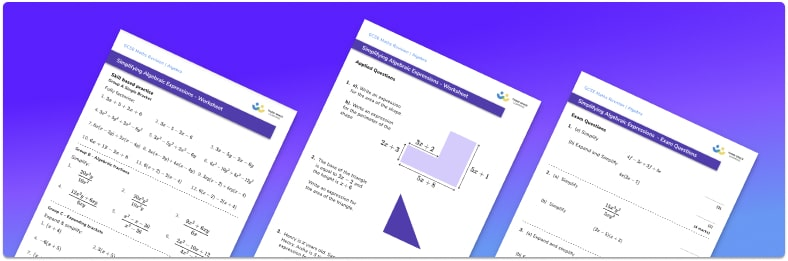• Section 1 of the algebraic expressions worksheet contains 30 skills-based algebraic expressions questions, in 3 groups to support differentiation
• Section 2 contains 3 applied algebraic expressions questions with a mix of worded problems and deeper problem solving questions
• Section 3 contains 4 foundation and higher level GCSE exam style algebraic expressions questions
• Answers and a mark scheme for all algebraic expressions questions are provided
• Questions follow variation theory with plenty of opportunities for students to work independently at their own level
• All questions created by fully qualified expert secondary maths teachers
• Suitable for GCSE maths revision for AQA, OCR and Edexcel exam boards

• This field is for validation purposes and should be left unchanged.

You can unsubscribe at any time (each email we send will contain an easy way to unsubscribe). To find out more about how we use your data, see our privacy policy.

### Algebraic expressions at a glance

Algebraic expressions are sets of terms with letters and numbers that are combined using addition, subtraction, multiplication and division. These can be binomial expressions (containing two terms), trinomial expressions (containing three terms) or other polynomial expressions. A monomial contains only one term.

In maths we are often asked to simplify algebraic expressions or to manipulate algebraic expressions.

To simplify algebraic expressions we need to collect like terms by looking at integer coefficients of terms with different exponents.

Manipulating algebraic expressions includes expanding brackets (sometimes called parentheses) by multiplication. This requires applying order of operations and using the distributive property.

Algebraic expressions also include factorising algebraic expressions, which is the reverse of expanding brackets. Factorising results in an expression being written as a product of factors.

Algebraic fractions are also a part of algebraic expressions so the skills we have for fractions such as addition, subtraction, simplifying and so on are extended to be used with algebra.

Looking forward, students can then progress to additional simplifying expressions worksheets and on to more algebra worksheets, for example a sequences worksheet, simultaneous equations worksheet or straight line graphs worksheet.For more teaching and learning support on Algebra our GCSE maths revision lessons provide step by step support for all GCSE maths concepts.

## Related worksheets

Simplifying Algebraic Fractions Worksheet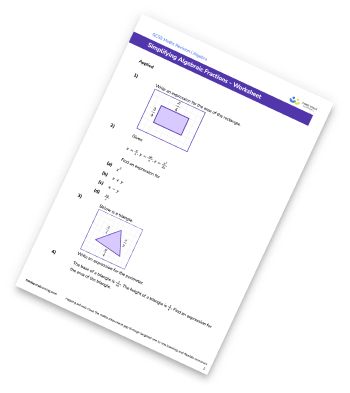Substitution Worksheet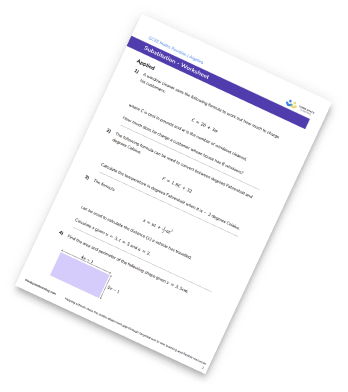Algebraic Fractions Worksheet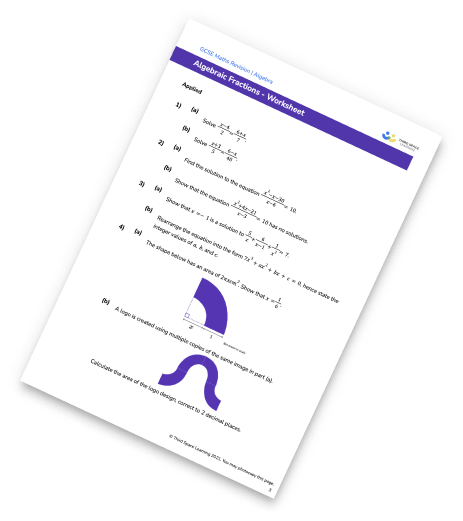Collecting Like Terms Worksheet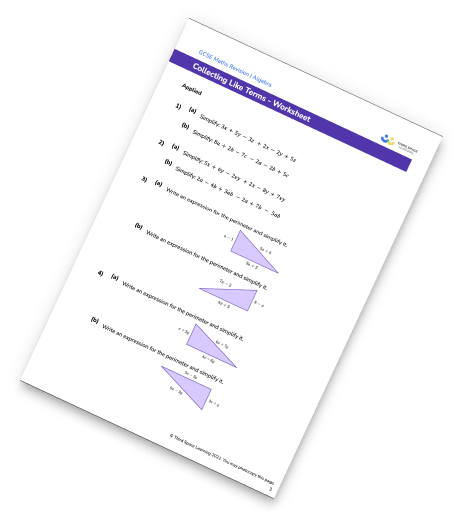## Do you have KS4 students who need more focused attention to succeed at GCSE?There will be students in your class who require individual attention to help them succeed in their maths GCSEs. In a class of 30, it’s not always easy to provide.

Help your students feel confident with exam-style questions and the strategies they’ll need to answer them correctly with our dedicated GCSE maths revision programme.

Lessons are selected to provide support where each student needs it most, and specially-trained GCSE maths tutors adapt the pitch and pace of each lesson. This ensures a personalised revision programme that raises grades and boosts confidence.

Find out more